Next: Energy of Gravity Waves Up: Waves in Incompressible Fluids Previous: Gravity Waves in Deep

# Gravity Waves in Shallow Water

Consider the so-called shallow water limit,(11.35)

in which the depth,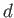, of the water is much less than the wavelength,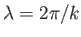, of the wave. In this limit, the gravity wave dispersion relation (11.21) reduces to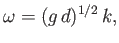(11.36)

because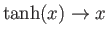as. It follows that the phase velocities and group velocities of gravity waves in shallow water all take the fixed value(11.37)

irrespective of wave number. We conclude that--unlike deep water waves--shallow water gravity waves are non-dispersive in nature (Fitzpatrick 2013). In other words, in shallow water, waves pulses and plane waves all propagate at the same speed. It can be seen that the velocity (11.37) increases with increasing water depth.

For a plane wave of wave number, in the limit, Equation (11.19) yields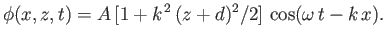(11.38)

Hence, Equations (11.7) and (11.27) give [cf., Equations (11.45)-(11.48)]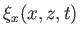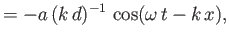(11.39)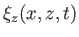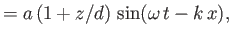(11.40)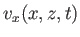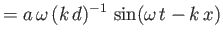(11.41)(11.42)

Here,is again the amplitude of the vertical oscillation at the water's surface. According to the previous expressions, the passage of a shallow water gravity wave causes a water particle located a depth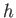below the surface to execute an elliptical orbit, of horizontal radius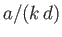, and vertical radius, about its equilibrium position. The orbit is greatly elongated in the horizontal direction. Furthermore, its vertical radius decreases linearly with increasing depth such that it becomes zero at the bottom (i.e., at). As before, whenever the particle's vertical displacement attains a maximum value the particle is moving horizontally in the same direction as the wave, and vice versa.Next: Energy of Gravity Waves Up: Waves in Incompressible Fluids Previous: Gravity Waves in Deep
Richard Fitzpatrick 2016-03-31